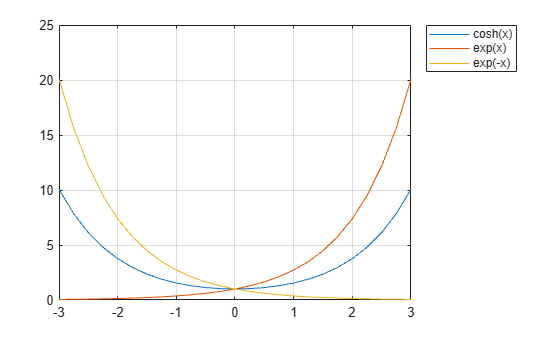Documentation

# cosh

Hyperbolic cosine

## Syntax

``Y = cosh(X)``

## Description

example

````Y = cosh(X)` returns the hyperbolic cosine of the elements of `X`. The `cosh` function operates element-wise on arrays. The function accepts both real and complex inputs. All angles are in radians.```

## Examples

collapse all

Create a vector and calculate the hyperbolic cosine of each value.

```X = [0 pi 2*pi 3*pi]; Y = cosh(X)```
```Y = 1×4 103 × 0.0010 0.0116 0.2677 6.1958 ```

Plot the hyperbolic cosine function over the domain $-5\le x\le 5.$

```x = -5:0.01:5; y = cosh(x); plot(x,y) grid on```The hyperbolic cosine satisfies the identity $\mathrm{cosh}\left(\mathit{x}\right)=\frac{{\mathit{e}}^{\mathit{x}}+{\mathit{e}}^{-\mathit{x}}}{2}$. In other words, $\mathrm{cosh}\left(\mathit{x}\right)$ is the average of ${\mathit{e}}^{\mathit{x}}$ and ${\mathit{e}}^{-\mathit{x}}$. Verify this by plotting the functions.

Create a vector of values between -3 and 3 with a step of 0.25. Calculate and plot the values of `cosh(x)`, `exp(x)`, and `exp(-x)`. As expected, the curve for `cosh(x)` lies between the two exponential curves.

```x = -3:0.25:3; y1 = cosh(x); y2 = exp(x); y3 = exp(-x); plot(x,y1,x,y2,x,y3) grid on legend('cosh(x)','exp(x)','exp(-x)','Location','bestoutside')```## Input Arguments

collapse all

Input angles in radians, specified as a scalar, vector, matrix, or multidimensional array.

Data Types: `single` | `double`
Complex Number Support: Yes

collapse all

### Hyperbolic Cosine

The hyperbolic cosine of an angle x can be expressed in terms of exponential functions as

`$\mathrm{cosh}\left(x\right)=\frac{{e}^{x}+{e}^{-x}}{2}.$`

In terms of the traditional cosine function with a complex argument, the identity is

`$\mathrm{cosh}\left(x\right)=\mathrm{cos}\left(ix\right)\text{\hspace{0.17em}}.$`